# 991 Results

View
Selected filters:
• Module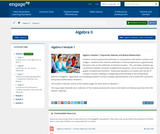Conditional Remix & Share Permitted
CC BY-NC-SA
Rating

Students connect polynomial arithmetic to computations with whole numbers and integers.  Students learn that the arithmetic of rational expressions is governed by the same rules as the arithmetic of rational numbers.  This unit helps students see connections between solutions to polynomial equations, zeros of polynomials, and graphs of polynomial functions.  Polynomial equations are solved over the set of complex numbers, leading to a beginning understanding of the fundamental theorem of algebra.  Application and modeling problems connect multiple representations and include both real world and purely mathematical situations.

Subject:
Algebra
Material Type:
Module
Provider:
New York State Education Department
Provider Set:
EngageNY
Date Added:
05/14/2013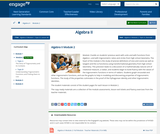Conditional Remix & Share Permitted
CC BY-NC-SA
Rating

Module 2 builds on students previous work with units and with functions from Algebra I, and with trigonometric ratios and circles from high school Geometry. The heart of the module is the study of precise definitions of sine and cosine (as well as tangent and the co-functions) using transformational geometry from high school Geometry. This precision leads to a discussion of a mathematically natural unit of rotational measure, a radian, and students begin to build fluency with the values of the trigonometric functions in terms of radians. Students graph sinusoidal and other trigonometric functions, and use the graphs to help in modeling and discovering properties of trigonometric functions. The study of the properties culminates in the proof of the Pythagorean identity and other trigonometric identities.

Subject:
Algebra
Material Type:
Module
Provider:
New York State Education Department
Provider Set:
EngageNY
Date Added:
08/15/2014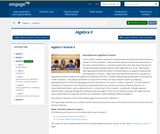Conditional Remix & Share Permitted
CC BY-NC-SA
Rating

In this module, students synthesize and generalize what they have learned about a variety of function families.  They extend the domain of exponential functions to the entire real line (N-RN.A.1) and then extend their work with these functions to include solving exponential equations with logarithms (F-LE.A.4).  They explore (with appropriate tools) the effects of transformations on graphs of exponential and logarithmic functions.  They notice that the transformations on a graph of a logarithmic function relate to the logarithmic properties (F-BF.B.3).  Students identify appropriate types of functions to model a situation.  They adjust parameters to improve the model, and they compare models by analyzing appropriateness of fit and making judgments about the domain over which a model is a good fit.  The description of modeling as, the process of choosing and using mathematics and statistics to analyze empirical situations, to understand them better, and to make decisions, is at the heart of this module.  In particular, through repeated opportunities in working through the modeling cycle (see page 61 of the CCLS), students acquire the insight that the same mathematical or statistical structure can sometimes model seemingly different situations.

Subject:
Algebra
Material Type:
Module
Provider:
New York State Education Department
Provider Set:
EngageNY
Date Added:
09/16/2014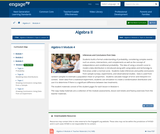Conditional Remix & Share Permitted
CC BY-NC-SA
Rating

Students build a formal understanding of probability, considering complex events such as unions, intersections, and complements as well as the concept of independence and conditional probability.  The idea of using a smooth curve to model a data distribution is introduced along with using tables and techonolgy to find areas under a normal curve.  Students make inferences and justify conclusions from sample surveys, experiments, and observational studies.  Data is used from random samples to estimate a population mean or proportion.  Students calculate margin of error and interpret it in context.  Given data from a statistical experiment, students use simulation to create a randomization distribution and use it to determine if there is a significant difference between two treatments.

Subject:
Algebra
Material Type:
Module
Provider:
New York State Education Department
Provider Set:
EngageNY
Date Added:
03/24/2016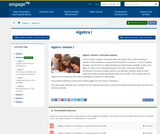Conditional Remix & Share Permitted
CC BY-NC-SA
Rating

In this module, students reconnect with and deepen their understanding of statistics and probability concepts first introduced in Grades 6, 7, and 8. Students develop a set of tools for understanding and interpreting variability in data, and begin to make more informed decisions from data. They work with data distributions of various shapes, centers, and spreads. Students build on their experience with bivariate quantitative data from Grade 8. This module sets the stage for more extensive work with sampling and inference in later grades.

Subject:
Statistics and Probability
Material Type:
Module
Provider:
New York State Education Department
Provider Set:
EngageNY
Date Added:
08/01/2013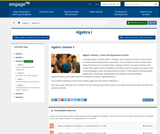Conditional Remix & Share Permitted
CC BY-NC-SA
Rating

In earlier grades, students define, evaluate, and compare functions and use them to model relationships between quantities. In this module, students extend their study of functions to include function notation and the concepts of domain and range. They explore many examples of functions and their graphs, focusing on the contrast between linear and exponential functions. They interpret functions given graphically, numerically, symbolically, and verbally; translate between representations; and understand the limitations of various representations.

Subject:
Algebra
Material Type:
Module
Provider:
New York State Education Department
Provider Set:
EngageNY
Date Added:
09/17/2013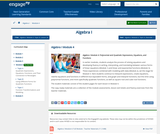Conditional Remix & Share Permitted
CC BY-NC-SA
Rating

In earlier modules, students analyze the process of solving equations and developing fluency in writing, interpreting, and translating between various forms of linear equations (Module 1) and linear and exponential functions (Module 3). These experiences combined with modeling with data (Module 2), set the stage for Module 4. Here students continue to interpret expressions, create equations, rewrite equations and functions in different but equivalent forms, and graph and interpret functions, but this time using polynomial functions, and more specifically quadratic functions, as well as square root and cube root functions.

Subject:
Algebra
Material Type:
Module
Provider:
New York State Education Department
Provider Set:
EngageNY
Date Added:
09/17/2013Unrestricted Use
CC BY
Rating

Material Type:
Module
Provider:
Rice University
Provider Set:
OpenStax College
Date Added:
09/20/2018Unrestricted Use
CC BY
Rating

Material Type:
Module
Provider:
Rice University
Provider Set:
OpenStax College
Date Added:
09/20/2018Unrestricted Use
CC BY
Rating

Material Type:
Module
Provider:
Rice University
Provider Set:
OpenStax College
Date Added:
09/20/2018Unrestricted Use
CC BY
Rating

Material Type:
Module
Provider:
Rice University
Provider Set:
OpenStax College
Date Added:
09/20/2018Unrestricted Use
CC BY
Rating

Material Type:
Module
Provider:
Rice University
Provider Set:
OpenStax College
Date Added:
09/20/2018Unrestricted Use
CC BY
Rating

Material Type:
Module
Provider:
Rice University
Provider Set:
OpenStax College
Date Added:
09/20/2018Unrestricted Use
CC BY
Rating

Material Type:
Module
Provider:
Rice University
Provider Set:
OpenStax College
Date Added:
09/20/2018Unrestricted Use
CC BY
Rating

Material Type:
Module
Provider:
Rice University
Provider Set:
OpenStax College
Date Added:
09/20/2018Unrestricted Use
CC BY
Rating

Material Type:
Module
Provider:
Rice University
Provider Set:
OpenStax College
Date Added:
09/20/2018Unrestricted Use
CC BY
Rating

Material Type:
Module
Provider:
Rice University
Provider Set:
OpenStax College
Date Added:
09/20/2018Unrestricted Use
CC BY
Rating

Material Type:
Module
Provider:
Rice University
Provider Set:
OpenStax College
Date Added:
09/20/2018Unrestricted Use
CC BY
Rating

Material Type:
Module
Provider:
Rice University
Provider Set:
OpenStax College
Date Added:
09/20/2018Unrestricted Use
CC BY
Rating

Material Type:
Module
Provider:
Rice University
Provider Set:
OpenStax College
Date Added:
09/20/2018